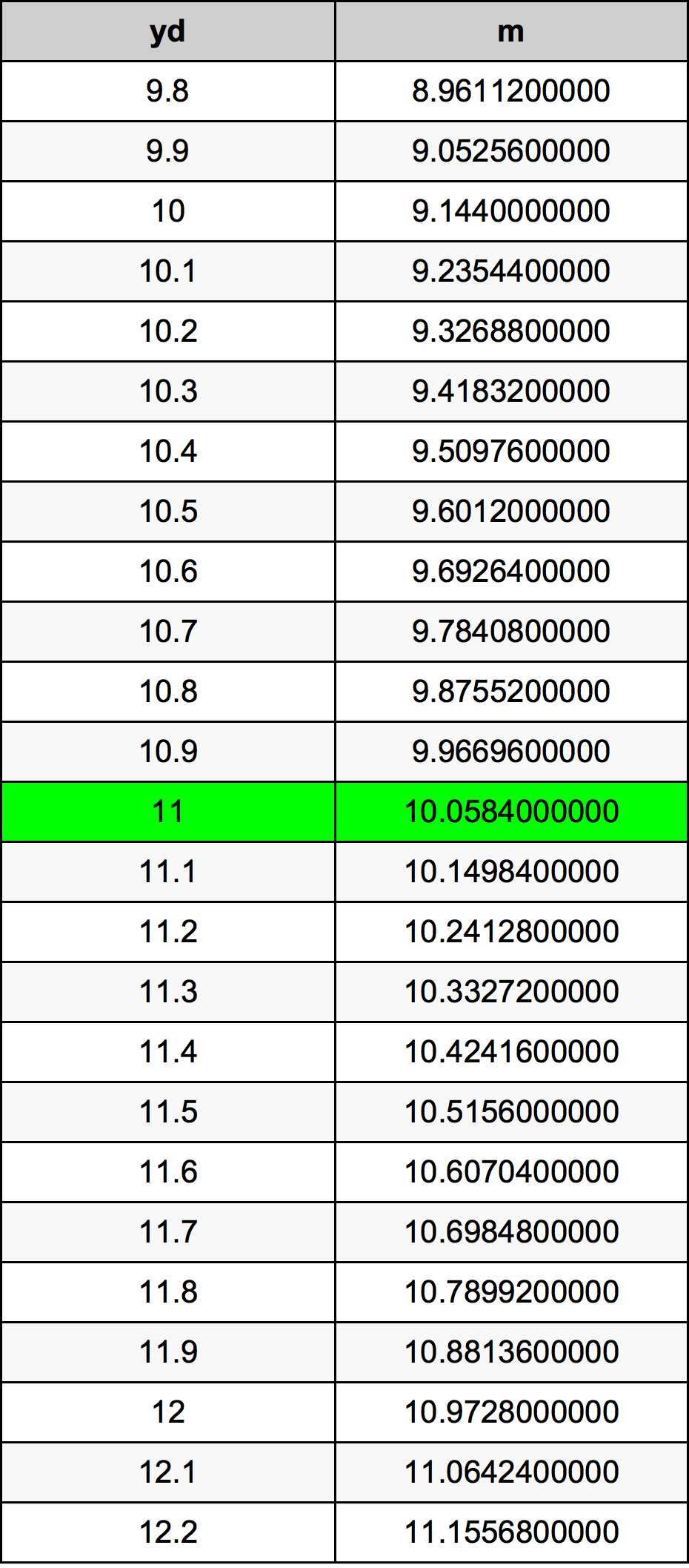Yards To Meters

# 11 yd to m11 Yards to Meters

yd
=
m

## How to convert 11 yards to meters?

 11 yd * 0.9144 m = 10.0584 m 1 yd
A common question is How many yard in 11 meter? And the answer is 12.0297462817 yd in 11 m. Likewise the question how many meter in 11 yard has the answer of 10.0584 m in 11 yd.

## How much are 11 yards in meters?

11 yards equal 10.0584 meters (11yd = 10.0584m). Converting 11 yd to m is easy. Simply use our calculator above, or apply the formula to change the length 11 yd to m.

## Convert 11 yd to common lengths

UnitLength
Nanometer10058400000.0 nm
Micrometer10058400.0 µm
Millimeter10058.4 mm
Centimeter1005.84 cm
Inch396.0 in
Foot33.0 ft
Yard11.0 yd
Meter10.0584 m
Kilometer0.0100584 km
Mile0.00625 mi
Nautical mile0.0054311015 nmi

## What is 11 yards in m?

To convert 11 yd to m multiply the length in yards by 0.9144. The 11 yd in m formula is [m] = 11 * 0.9144. Thus, for 11 yards in meter we get 10.0584 m.

## 11 Yard Conversion Table## Alternative spelling

11 Yards to Meter, 11 Yards in Meter, 11 Yards to m, 11 Yards in m, 11 Yard to Meters, 11 Yard in Meters, 11 yd to Meter, 11 yd in Meter, 11 Yards to Meters, 11 Yards in Meters, 11 Yard to Meter, 11 Yard in Meter, 11 yd to m, 11 yd in m